Tetration Gottfried Helms - Univ Kassel          07' 2007 - 2008# An iterative procedure for Pari/GP (pseudocode)

Version 2. Some changes in formulas were made for convenience, which resulted in different signs-convention for the A-matrices and denominators (no overall effect of changes)

Gottfried Helms,11.1.2010
first version: 9.8.2008

## Intro

The reason for the computation of the eigendecomposition of the Ut-matrices is, that fractional iterates of Ut-tetration (x->tx -1) can be computed using fractional powers of the matrix(-operator) Ut.

This concept is not new; it is known in the context of Bell- and Carleman-matrices for iteration of functions. However, the diagonalization method is only one nethod to make use of these matrices to arrive at fractional iteration as meaningful interpolation of the integer iteration. Another method involves the use of the binomial expansion instead.

The general question of a method-independent, external appropriateness-criterion for the selection of an interpolation-method for fractional iteration of the exponential-function (expb(x)) or of the "decremented exponential-function" (dxpt(x) or Ut(x)as I call it here) is not yet answered and the decision for the "unique" appropriate interpolation-method is thus still open.

An "external" argument could be, that the interpolations for fractional iterates should be invariant across different fixpoint-shifts. I should explain this a bit.

Assume the usual tetrational function

Tb(x) = bx
Tb°1(x)  = Tb(x)
Tb°h(x) = Tb°h-1(Tb(x))

then Tb(x)has inifinitely many fixpoints t0,t1,t2,... (almost all are complex), such that

Tb°h(tk) = tk      or: btk = tk        or:   b = tk1/tk

Then we may translate the problem

Tboh(x) = (Utkoh(x/tk – 1 ) +1)*tk .

The advantage of this fixpoint-shift is then, that the powerseries for Utk(x) has no constant term (then matrix-operator for Utk() is triangular) such that it can be –for instance – inverted. Also fractional iterates can then formally be computed using matrix-logarithm or diagonalization.

The problem with this is that it is possible (there was made an argument in a discussion), that these fixpoint-shifts give different results using different fixpoints. If this is indeed the case, then a unique definition of tetration must include the convention which fixpoint has to be taken (besides of the question, whether this method is appropriate at all for the complete mathematical context).

However; the matrix-based methods and also diagonalization has been, and is, in use in the research, so I leave this problem open and I'm going to discuss the method for the cases where no fixpoint-shift is needed further to exhibit some deeper details.

The diagonalization gives consistent results in the sense that, for instance,

Uto0.5(Uto0.5(x)) = Uto0.5+0.5(x) = Uto1(x)

or more generally

Utoa(Utob(x)) = Utoa+b(x) .

as far as always the same method is applied.

Here I give technical details, how to compute the diagonalization matrices (eigen-matrices) for such triangular matrix-operators and some sample values for comparision, which I haven't seen elsewhere.

Further links: a more detailed description can be found at

A different approach, based on polynomial interpolation which however gives the same result can be found at

For an intro in tetration see also ; a list of valuable references can be found in  and .

## Eigendecomposition and Schröder-function

If we denote

V(x)~ * Uth = V(Utoh(x))~

(see all matrix-definitions in appendix 1) then fractional powers of Ut can be computed by fractional powers of the diagonal matrix D of the eigen-decomposition of Ut:

V(x)~ * Uth = V(x)~ * (W * Dh*W-1) =  V(Utoh(x))~

where D is the diagonal-matrix of eigenvalues and W is the triangular matrix of eigenvectors. (Note, that W and W-1 are only unique besides column-scaling of W if they are assumed to be triangular)

In my computations I use a column-normed version of W having unit-diagonal; then W seems to be also a matrix-operator. It provides then the coefficients of the Schröder-function σ which decomposes the iteration of a function into

Utoh(x) = σ-1(uh*σ(x))

(see a short definition of the Schröder-function at (superlogarithm); Schröders article at )

In the light of Schröder's result, that

limh->infh(x)/f'(0)h = σ(x)

which is also

limh->infh(x)/uh = σ(x)

and where we find the relevant 2'nd column of the matrix W just being that limit, the assumed property, that W is in fact a matrix-operator, is then evident.

The matrix D is diagonal, containing the consecutive powers of u = log(t).

Note: the given eigen-decomposition can only be computed, if t≠exp(1), u≠1, so we discuss only iterations of Ut(x) = tx –1 ≠  exp(x) – 1here.

## The Pari/GP-pseudocode

Besides numerical matrices Pari/GP can handle symbolic coefficients in a basic way, so we can feed the following procedure with the Ut-matrix containing u as unevaluated symbol as well to get the symbolic solution. However, the symbolic description of terms is much consumptive with increasing index; matrix-size of 16x16 gives the result in a blink; 32x32 needs a bit of time, for 64x64 matrices computation time and memory requirements are already excessive (see a zoomed image coefficients-matrix at a16 in appendix 2)

The input for the following procedure is some triangular matrix (here Ut, where u=log(t)≠1 ). The matrices W and W-1 are initialized with the unit-diagonal and then new entries are computed using the previously computed ones. Here in W we can compute the columns and in W-1 the rows separately, always beginning at the diagonalelement (which is 1) proceeding to the element at position r,c vertically resp. horizontally right-to-left.

 { TRI_EW(U) =         local(u=U[2,2], W, D, Wi, t=exp(u));   dim=rows(U);   D=diag(U); \\ for operator-matrices Ut this is 1,u,u2,u3,... W=matid(dim);   for(c=1,dim-1,      for(r=c+1,dim,        Wr,c=sum(k=c,r-1,Ur,k*Wk,c)  / (Dc-Dr) ))Wi=matid(dim); \\ means matrix W-1   for(r=2,dim,        forstep(c=r-1,1,-1,          Wir,c=sum(k=0,r-1-c,Wir,r-k*Ur-k,c) / (Dr-Dc) ))return([[u,t,exp(u/t)],W,D,Wi]); }

## Coefficients of the powerseries for fractional iterates

Then the powerseries for Ut(x) = tx-1 and its h'th iterate Ut°h(x) due to the eigendecomposition

Uth = W * dV(uh) * W-1

is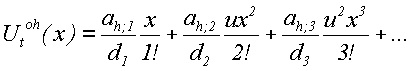The denominators dk are products

 dk =The ah;k are polynomials in u and uh; in the following the ah;k are expressed as matrices of coefficients Ak of these polynomials:

 A1 ==> ah,1= 1*uh d1 = 1 A2 ==> ah;2 = (1* uh – 1*u2h) d2= (u-1) A3 ==> ah;3     = (1* uh – 3*u2h+ 2*u3h)                     + u(2* uh – 3*u2h+ 1*u3h)                  =    (1 + 2u)  uh                      – (3 + 3u) u2h                      + (2 + 1u) u3h   d3= (u-1)(u2-1) A4 =d4= (u-1)(u2-1)(u3-1) A5 =A6 =A7 =A8 =The number of rows at ah;k are binomial(k-1,2)+1, the number of columns is k+1.

The highest exponent to which a power of u appears is thus binomial(k-1,2) due to the rows plus k*h due to the columns.

For instance in ah;8 for height h=1 is thus (binomial(7,2))+8*h=21+8 = 29 and for h=2 is 37.

However, for the complete coefficient at xk this is increased by the coefficient uk-1 and also decreased by exponents of u in the denominator d8; here the highest exponent is binomial(k,2)=28 so the remaining most significant power of u at x8 is u21+8*h+(8-1)-28 which is u8 for h=1 and u16 for h=2 . In general, the ratio of most significant powers of u in numerator and denominator for each k is simply uk*h .

If u is in the near of 1, and h is fractional, then additional hyperbolic effects occur since the denominators approach zero if integer powers un approach 1, and these are not evenly cancelled by the numerators if h is noninteger.

For integer heights the coefficients ah;k are evenly divisible by the denominators and only few different powers of u are involved in the final coefficient ah;k/dk; for fractional h this is not the case. In that case we have a much more complicated structure of powers of u (however the most significant power is still uk*h.)

The explicite computation of the coefficients ak is

 ah;k = V(u)~*Ak*V(uh)=where the index of matrices are beginning at 1 as used in Pari/GP

## The coefficients-matrices for heights 1 and 2

Heights h introduce higher powers of u into the coefficients. For the coefficients-matrices Ak this means an increasing rowshift of each column according to height h. Let now D denote the coefficients at powers of u of the denominator, which are independent of the height-parameter. Then if the Ak matrices are rewritten according to integer heights, they occur to be linear combinations of (shifted) D-vectors. This shifting expresses multiplications with powers of u.

The rows of the following matrices agree to the resp. powers of u, so I added the accordingly vectors of u-powers in the most-left column to make this explicit. Since the row-shifting "renormalized" the powers of u depending on the h-parameter, we can simply use the row-sums to arrive at the final values for the scalar coefficient ah,k.

 A4 dep. on h rowsums decomposition into multiples of D4 ah,k =~ *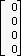= V(u)~ * D4 * 0~*= u * V(u)~ * D4 *~*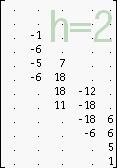= u2 *V(u)~ * D4 *[1, 7u, 6u2, 1u3]

## The coefficients for the Schröder-function

The coefficients of the powerseries for the Schröder-function for Ut(x) ,  σUt , occur in the second column of W, so that

V(x)~ * W = V(σUt(x))~

and for the inverse

V(x)~ * W-1 = V(σUt-1(x))~

so that finally

V(x)~               * W                 = V(σUt(x))~
V(σUt(x))~        * dV(uh)           = V(uh * σUt(x))~
V(uh * σUt(x))~  * W-1              = V(σUt-1(uh σUt(x)))~
= V(Ut°h(x))~

The powerseries isThe denominators dk are the same as the in the previous

 dk =The sk are polynomials in u only; in the following they are expressed as rowvectors of their coefficients (Sk ), where the leftmost entry is associated with u0:

 S1 ==> s1= 1 d1 = 1 S2 ==> s2 = 1 d2= (u-1)/u S3 ==> s3     = (1 + 2u)  d3=  (u-1)(u2-1)/u2 S4 =S5 =S6 =S7 =The number of columns at sk are binomial(k-1,2)+1, so the highest exponent to which a power of u appears in sk is thus binomial(k-1,2).

Note, that these coefficients occur also in the corresponding A-matrices in the second column, which agrees to the fact, that the schröder-function is assumed to be the limit of the case h->inf: then all other columns of that A-matrices get shifted to infinity and –if |u|<1 – vanish by approximation of uh to zero.

We have from this

 sk = Sk *V(u) =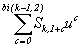To see the ratio of the highest exponent of u in numerator and denominator we add binomial(k-1,2)+1 and (k-1) according to the main formula and subtract binomial(k,2) according to the denominator which comes out to be zero for each k.

The coefficients for the inverse of the Schröder-function are in the same way taken from W-1 instead.

## Appendix 1: Definitions for matrix-notation

For U-tetration the matrices U and Utare the triangular Stirling-matrix scaled as the following:

U = dF-1*St2 * dF                      // performs x - >exp(x)-1
Ut = dV(u)* U                          // where u=log(t); performs x -> tx – 1

where the involved (infinite size assumed) matrices are (only the top-left segment is displayed):

 V(u) =             dV(u) = diag(V(u)) columnvector([1, u, u2,u3,u4,...]) diagonal arrangement dF = diag(0!,1!,2!,3!,...) St2 =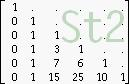Stirlingnumbers 2nd kind dF-1*St2 * dF = U =Ut =the numerical value for u=log(t) is actually used here

Note: the related Bell-matrix is just another convention to use Ut: it is a similarity-scaling of Ut, where the factorials are removed

BELLt = dV(u) * St2

The Carleman-matrix is the transposed of the Bell-matrix.

## Appendix 2: Coefficient-matrix A16 for a16

Here is a zoomed image of the coefficient-matrix A16 for a16 at x16:Gottfried Helms; 9. Aug. 2008

11.1.2010: added info about identity of W-column and A-matrix (in context to schröder-function)

Further references:

 wikipedia : "tetration", superlogarithm

 Andrew Robbins, "home of tetration" references in article

 Ioannis Galidakis, section "Lambert-W, tetration..." references

The article of

 Ernst Schröder, "Über iterirte Funktionen"; in: Mathematische Annalen, Band 3, Heft 296, 1871